# Network Theory – Introduction and Review

By on

In electrical engineering, Network Theory is the study of how to solve circuit problems.  By analyzing circuits, the engineer looks to determine the various voltages can currents with exist within the network.

When looking at solving any circuit, a number of methods and theories exist to assist and simplify the process.  This post briefly lists some of the more common network theories. For more detailed analysis of any particular theory you can search the myElectrical site or find more information on one of the many other sources available on the web.

Typically in network theory, we deal with linear and passive elements – most commonly:

• resistors add resistance, R to a circuit.  The inverse of resistance (1/R) is the conductance, G.
• capacitors add capacitance, C to a circuit.
• inductors add inductance, L to a circuit.
• reactance, X is a product of having capacitance or inductance in an alternating current (ac) circuit.  The inverse of reactance (1/X) is the susceptance, B.
• impedance, Z is the combination of resistance and reactance in an ac circuit. The invers of impedance (1/Z) is the admittance, Y.
• voltage source is a source of voltage within the circuit.  An ideal voltage source contains no internal series resistance.
• current source is a source of current within a circuit. An ideal current source contains no internal parallel resistance.

## Network Theories

### Series and Parallel Circuits

The connecting of elements in series or parallel is probably the most basic type of network.  In a series circuit the total resistance (or impedance) is the sum or resistances (or impedances).  In a parallel the inverse of the total resistance (or impedance) is the sum of the inverses.

The voltage in a series circuit is the some of voltages across each element, while the current is the same through each element.  In a parallel circuit the voltage is the same across each element, whilst the current is inversely proportional to the resistance (or impedance) of each circuit.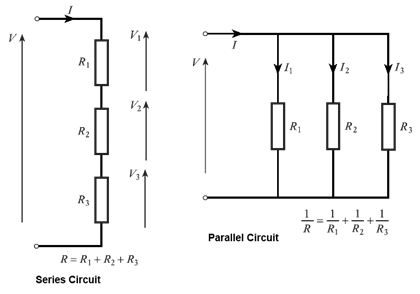Inductors follow the same law as resistors.  That is in series the total inductance is the sum of individual inductances, while in parallel the inverse of the total is the sum of the inverses.

Capacitors are the opposite.  In a series circuit the invers of total capacitance is the sum of the inverses for each individual capacitor.  For a parallel circuit the total capacitance is the sum of the individual capacitances.

Series and Parallel Circuits – Wikipedia page should you require a more detailed explanation

### Ohm's Law

Ohm's Law (developed in 1827 by Georg Ohm), states that the relationship given by the Voltage in a circuit divided by the current is a constant – the resistance (or impedance in ac circuits).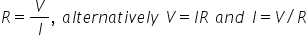Back to Basics - Ohms Law – myElectrical article explaining Ohm's Law

How Electrical Circuits Work – myElectrical introductory post with some Ohm's Law

### Kirchhoff's Laws

Kirchhoff's Laws comprise of two theorems – the current law and voltage law:

First Law (current) – the sum of currents at a circuit node equals zero

Second Law (voltage) – the sum of voltages around a loop equals zero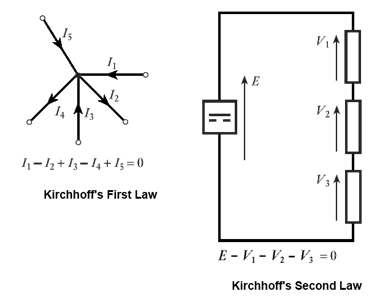Kirchhoff's circuit laws - Wikipedia page should you require a more detailed explanation

### Tellegen's Theorem

Tellegen's theorem simply states that the sum of power in all branches of a network is equal to zero, provided at network also complies with Kirchhoff's Laws. For a network with b, branches: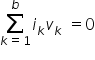This theorem is still relatively unknown, although it can be applied to a wide variety of problems, can form the basis for proof of most other network theories and applies to any type of element (passive,active, linear and non-linear).

Tellegen's theorem - Wikipedia page should you require a more detailed explanation

### Thévenin's Theorem

Thévenin's theorem states that any combination of network elements can be represented by a single voltage source and series resistor.  The equivalent voltage is calculated by having the branch open circuit; while the resistor is calculated by having any voltage sources short circuited;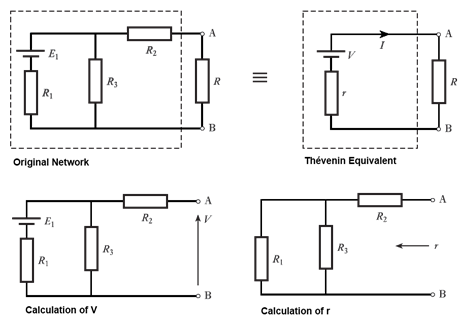Thévenin's theorem - Wikipedia page should you require a more detailed explanation

### Norton's Theorem

Norton's theorem states that any combination of network elements can be represented by a single current source and parallel resistor. The equivalent current is calculated by having the branch short circuited; while the resistor is calculated by having any voltage sources short circuited;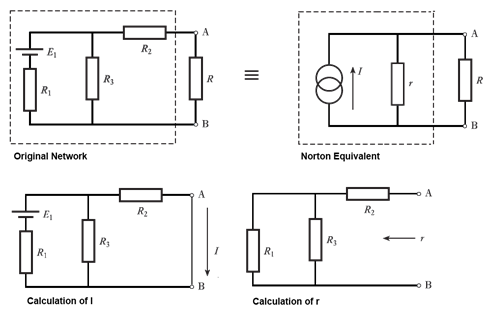Norton's theorem - Wikipedia page should you require a more detailed explanation

### Source Conversions

A constant voltage source is an ideal voltage in series with a resistor.  A constant  current source is an ideal current in parallel with a resistor.  To convert a voltage source to a current source or (vies-versa):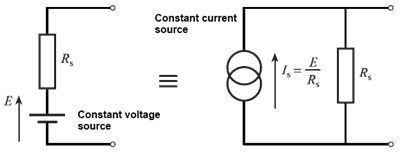Note: Norton's theorem could be stated as being Thévenin's theorem but with the equivalent current source

### Millman's Theorem

Millman's theorem applies to networks which contain only parallel branches and is used to find the voltage across all the branches.  If there are b, branches and each branch contains a resistor R and optional voltage E, than the branch voltage V, is given by:Millman's Theorem – allaboutcircuits.com worked example on the theory

### Star Delta Transforms

Star delta transforms are frequency used to convert between star (or PI) and delta network configurations: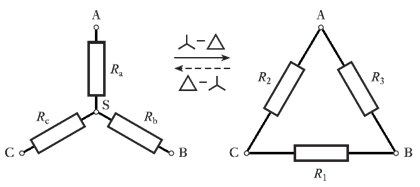Y-Δ transform - Wikipedia page should you require a more detailed explanation

### Nodal Analysis

Nodal analysis is a voltage method which relies on Kirchhoff's first law to produce a set of equations which can then be solved.  As a procedural method the steps involved are:

1. arbitrarily assign voltage to each node (V1, V2, etc.)
2. calculate the current in each branch, for example:  node 1 to node 2, resistance R12
gives, I12 = (V1-V2) / R12
3. at each node apply Kirchhoff's first law (sum of currents equals zero)
4. solve the resulting equations to find the voltages at each node (and hence currents)

Nodal analysis - Wikipedia page should you require a more detailed explanation

### Mesh Analysis

Mesh analysis is a current method which relies on Kirchhoff's second law to produce a set of equations which can then be solved.  As a procedural method the steps involved are:

1. assign a mesh (loop) current to each closed loop in the network
2. for each loop apply Kirchhoff's second law (sum of voltages equals zero)
3. solve the resulting equations to find the loop currents (and hence network voltages)

Mesh analysis - Wikipedia page should you require a more detailed explanation

### Superposition Theorem

If the network contains more than one voltage or current source, superposition allows for a solution to be found by considering each voltage or current source separately and then adding the results. To consider each source separately, all other voltage sources are short circuited and all other current sources are open circuited.

Note: superposition can be particular useful in deriving Thévenin or Norton equivalent networks.

Superposition Theorem - allaboutcircuits.com worked example on the theory

Author's Note:

I've put together the post as more of a summary and included links to other more detailed posts on the site were possible.  Where there is not a detailed post on the site, I have included links to articles on external sites so that our readers can still follow up.

While the external sites are good, it would be better if we had some myElectrical written content for the missing network theories.  If you're able to put together a post on one of these theorems to help fill this gaps, please do so – it will help greatly.

More interesting Notes:Steven McFadyen

Steven has over twenty five years experience working on some of the largest construction projects. He has a deep technical understanding of electrical engineering and is keen to share this knowledge. About the author

#### View 1 Comments (old system)

1.Notes says:
3/27/2013 1:51 PM

Per unit fault calculations is a method whereby system impedances and quantities are normalised across different voltage levels to a common base.  By removing the impact of varying voltages, the necessary calculations are simplified.  ... ...

Comments are closed for this post:
• have a question or need help, please use our Questions Section
• spotted an error or have additional info that you think should be in this post, feel free to Contact Us

How to Write an Electrical Note

Electrical notes are a collaborative collection of electrical engineering information and educational material. Any registered user can add content. ...

Differential protection, the good old days

This morning I was explaining how differential protection works to a junior engineer. To give him something to read I opened up the NPAG (Network Protection...

Low Voltage Fault Tables

The following tables provide quick order of magnitude fault levels for a a range of typical low voltage situations.

What is an Open Delta Transformer

In three phase systems, the use of transformers with three windings (or legs) per side is common.  These three windings are often connected in delta or...

What happened to the cable notes?

If you are wondering what happened to our cable notes, the short answer is that we have moved them to myCableEngineering.com.  The "Knowledge Base" at...

Fault Calculations - Introduction

Fault calculations are one of the most common types of calculation carried out during the design and analysis of electrical systems. These calculations...

Questions - Reputation and Privilege

Our question and answer system while letting you do exactly what it says, is much more.  It is a dynamic user driven system, where our users not only ask...

What is Aircraft Ground Power

Ever wondered what kind of power an aircraft uses when parked at the airport stand. Normally the aircraft generates it own power, but when parked with...

Equipment Verification (to IEC Standards)

One of the requirements to ensuring that everything works is to have equipment selected, manufactured and verified [tested] to IEC standards. Not all equipment...

Years ago I was told that you should always try to write the best report you can.  Many years later I still think on this as one of the better pieces of...## Have some knowledge to share

If you have some expert knowledge or experience, why not consider sharing this with our community.

By writing an electrical note, you will be educating our users and at the same time promoting your expertise within the engineering community.

To get started and understand our policy, you can read our How to Write an Electrical Note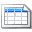Click here to view the notes list in a table formatClick here to view the notes indexed by tag